Several libraries are needed here. FRED database has a specific API through R to access the datasets: alfred. All other libraries are used for post-download purposes: manipulation, cleaning, plotting, etc.

library(alfred)
library(dplyr)
library(tidyr)
library(ggplot2)
library(ggthemes)
library(gganimate)
library(ggExtra)
library(DT)
library(skimr)
library(stringr)
library(lubridate)
library(writexl)
library(haven)

## FRED blog

FRED blog is an excellent resource for analyzing modern economic facts. Visit its recent story about unemployment in USA.

First, we look at average length (in weeks) of unemployment.

unemp_ave <- get_fred_series("UEMPMEAN")
datatable(unemp_ave)
skim(unemp_ave$UEMPMEAN) ## ## Skim summary statistics ## ## ── Variable type:numeric ─────────────────────────────────────────────────────────── ## variable missing complete n mean sd p0 p25 p50 p75 p100 ## unemp_ave$UEMPMEAN       0      850 850   16 7.2 7.1 11.6 14.1 17.6 40.7
##      hist
##  ▅▇▅▁▁▁▁▁

So, in three quick steps we:

2. Explore and view the data with datatable
3. Examine the descriptive statistics with skim

And finally, let’s see the time series in a plot:

ggplot(unemp_ave) +
geom_line(aes(x = date, y = UEMPMEAN))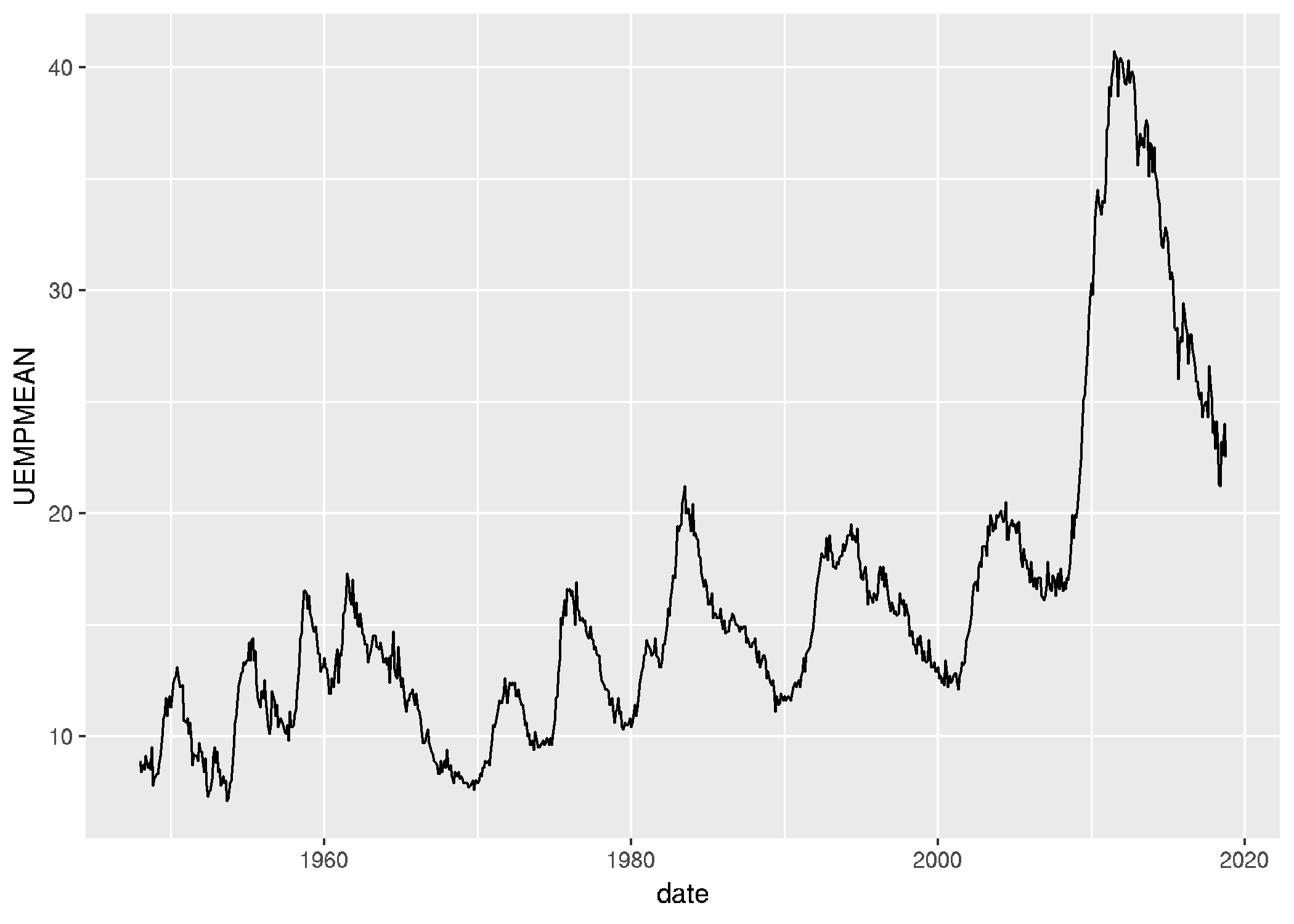## Unemployment rate

Now wen see the unemployment rate:

unemp_rate <- get_fred_series("UNRATE")

datatable(unemp_rate)
skim(unemp_rate$UNRATE) ## ## Skim summary statistics ## ## ── Variable type:numeric ─────────────────────────────────────────────────────────── ## variable missing complete n mean sd p0 p25 p50 p75 p100 ## unemp_rate$UNRATE       0      850 850 5.77 1.64 2.5 4.6 5.6 6.8 10.8
##      hist
##  ▂▅▇▆▅▂▁▁
ggplot(unemp_rate) +
geom_line(aes(x = date, y = UNRATE))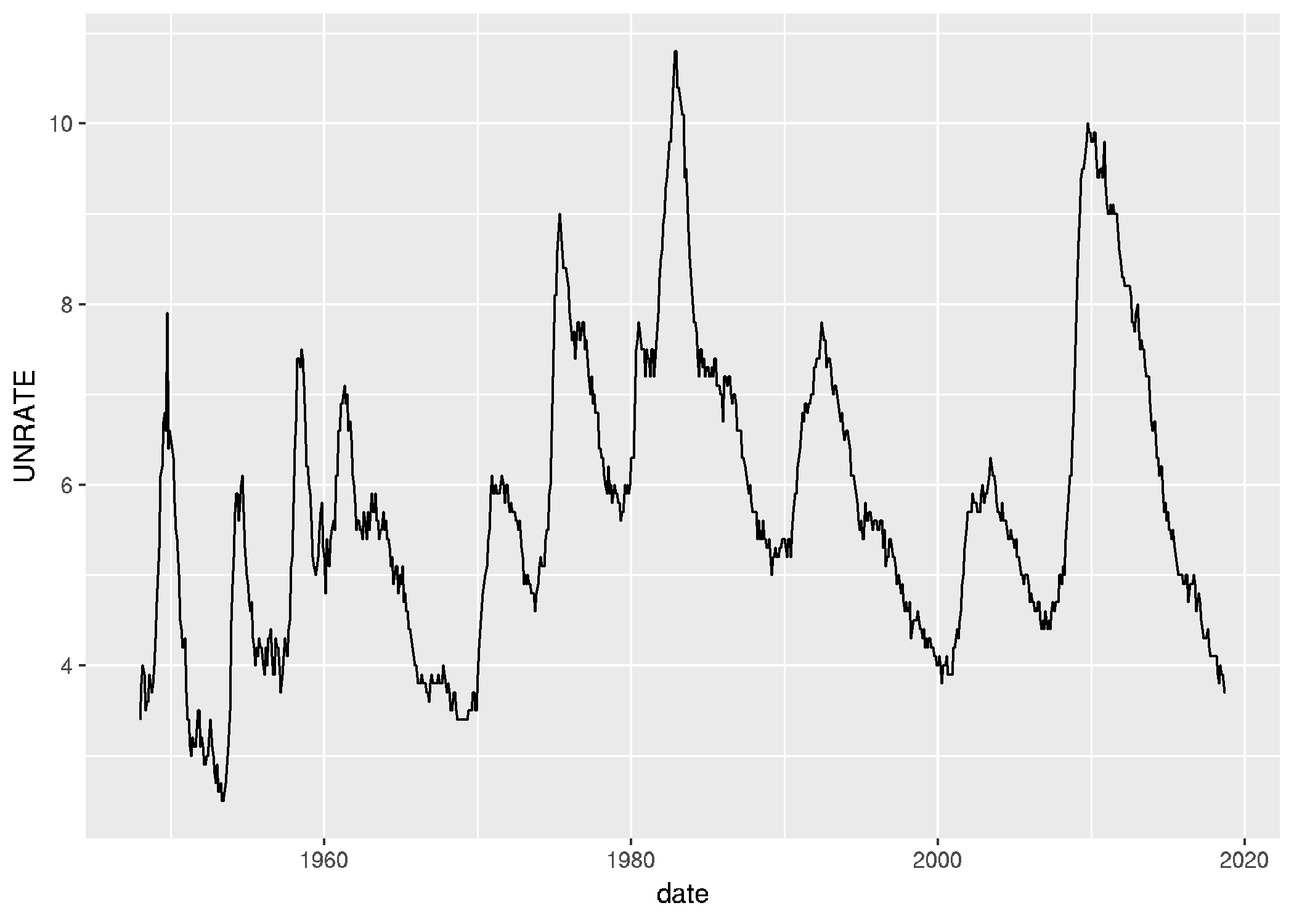And also produce a histogram that I would like to discuss with you:

ggplot(unemp_rate, aes(x = UNRATE)) +
geom_histogram(binwidth = 0.5, fill="blue", alpha=0.5, color="blue") +
theme_economist()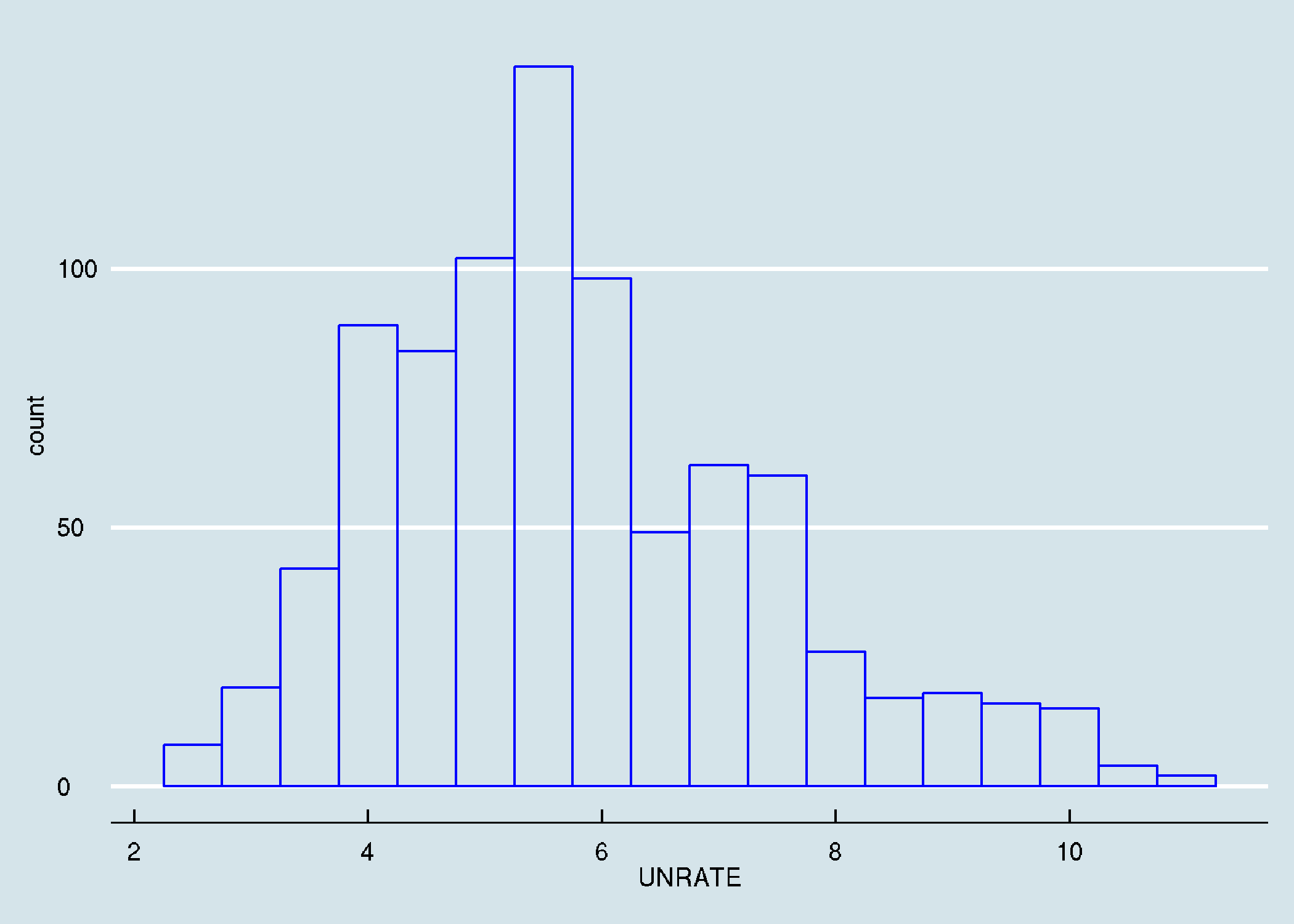We can even add more staff to the histogram:

ggplot(unemp_rate, aes(x = UNRATE)) +
geom_histogram(aes(y = ..density..), binwidth = 0.5, fill="steelblue", alpha=0.5, colour="blue") +
geom_line(stat="density", color="red", size = 1.5) +
stat_function(fun = dnorm, colour = "green", size = 1.5,
args = list(mean = mean(unemp_rate$UNRATE), sd = sd(unemp_rate$UNRATE))) +
theme_bw()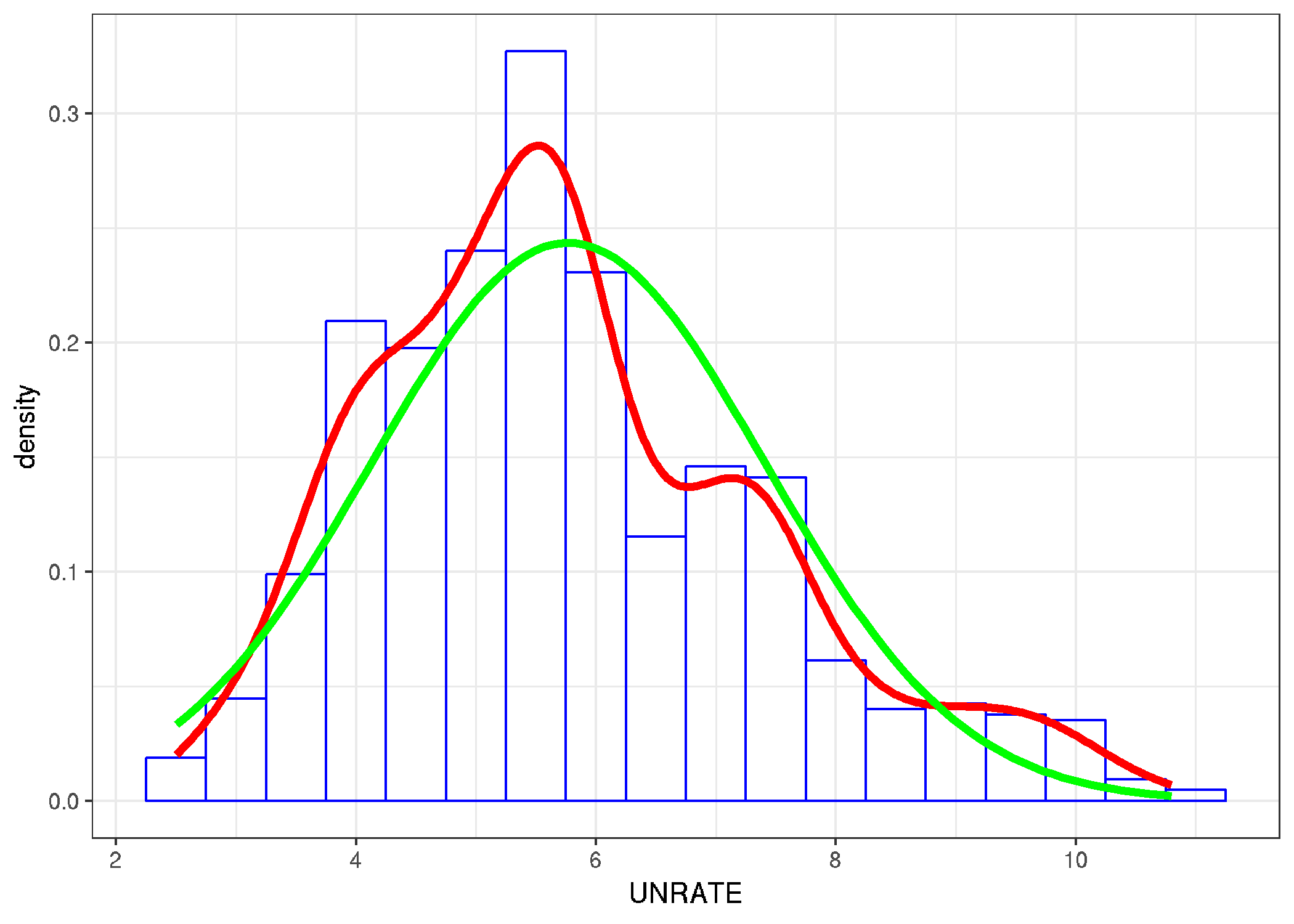## Unemployement by length

# Of Total Unemployed, Percent Unemployed 27 Weeks and over
unemp_05 <- get_fred_series("LNS13008397")

# Of Total Unemployed, Percent Unemployed 5 to 14 Weeks
unemp_05_14 <- get_fred_series("LNS13025701")

# Of Total Unemployed, Percent Unemployed 15 to 26 Weeks
unemp_15_26 <- get_fred_series("LNS13025702")

# Of Total Unemployed, Percent Unemployed 27 Weeks and over
unemp_27 <- get_fred_series("LNS13025703") 

Join the data sets

USA_unemp <- unemp_05 %>%
inner_join(unemp_05_14, by = "date") %>%
inner_join(unemp_15_26, by = "date") %>%
inner_join(unemp_27, by = "date") %>%
as_data_frame() %>%
rename(un_05    = LNS13008397,
un_05_14 = LNS13025701,
un_15_26 = LNS13025702,
un_27    = LNS13025703) %>%
select(date, un_05_14, un_15_26, un_27, un_05)

Transform the dataset and make the plot:

USA_unemp_gtr <- USA_unemp %>%
gather(unemp_type, value, -date)

gg.unemp <- USA_unemp_gtr %>%
ggplot(aes(x = date, y = value, fill = unemp_type)) +
geom_area(position = 'fill', aes(x=date, y=value)) +
theme_bw() +
labs(x = "Time", y = "Unempoyment share") +
theme(text = element_text(size = 14)) #+
#transition_reveal(unemp_type, date)

gg.unemp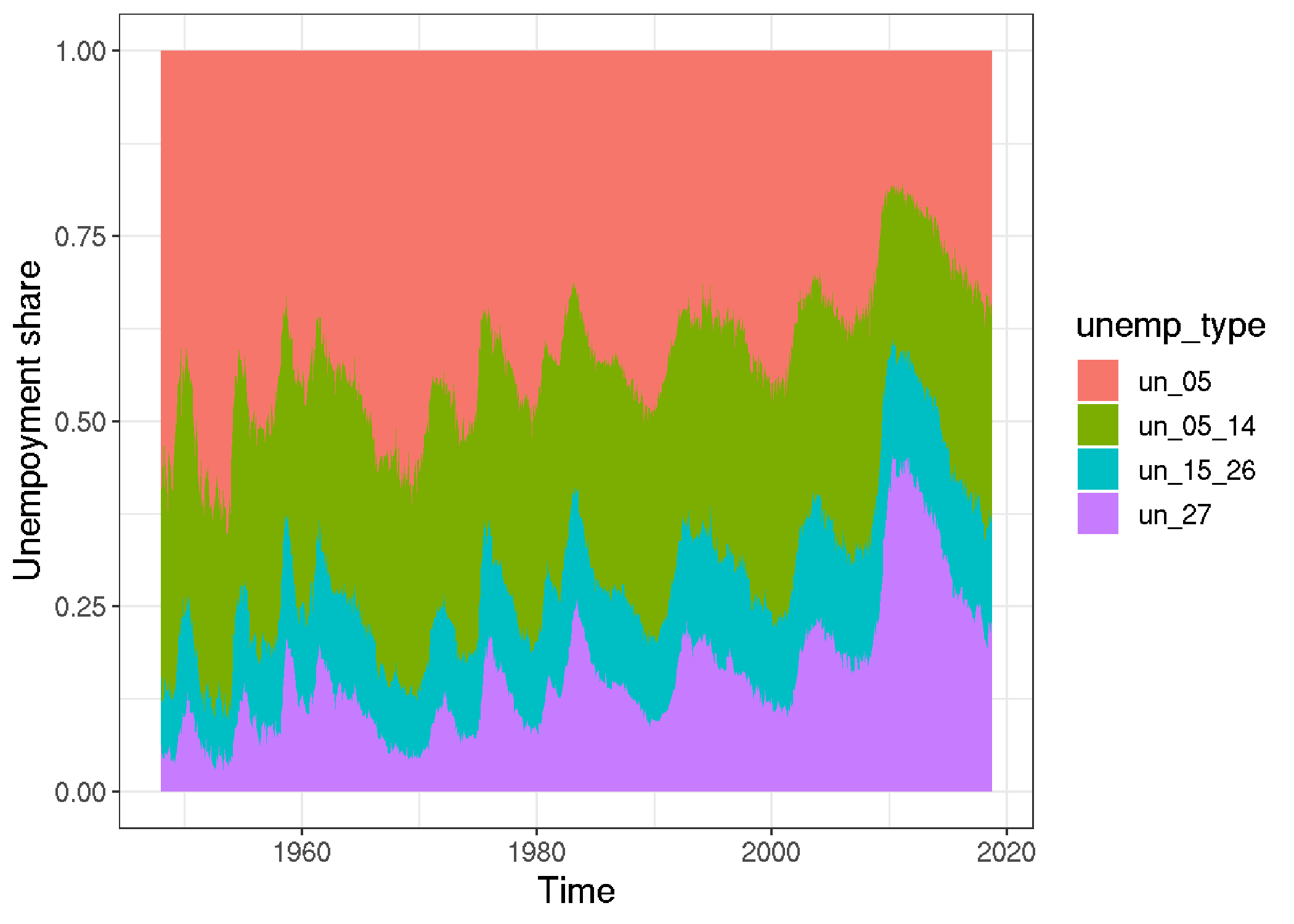#animate(gg.unemp, width = 960, height = 540, dpi = 300)